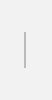# Standard deviation

## Explanation

A value that expresses the extent of variation of plural data. For example, in the case that all students who took a test got the same score, the standard deviation is zero (i.e., no variation). If there is a difference between the scores, the standard deviation takes a value greater than zero. Moreover, as the value increases, the variation gets bigger.
To obtain the standard deviation, average value of all data first be calculated. The values obtained by subtracting that average value from each data are then raised to the power of two. The sum of these values is then divided by the number of data, and the standard deviation is attained as the square root of that value.